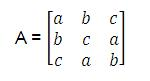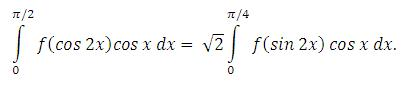×#### Thank you for registering.

One of our academic counsellors will contact you within 1 working day.

Click to Chat

1800-1023-196

+91-120-4616500

CART 0

• 0

MY CART (5)

Use Coupon: CART20 and get 20% off on all online Study Material

ITEM
DETAILS
MRP
DISCOUNT
FINAL PRICE
Total Price: Rs.

There are no items in this cart.
Continue Shopping• Complete JEE Main/Advanced Course and Test Series
• OFFERED PRICE: Rs. 15,900
• View Details

```IIT-JEE-Mathematics-Mains-2003

MAINS
_______________________________________________________________

1. If z1 and z2 are two complex numbers such that

|z1| < 1 < |z1|then prove that2. Find a point on the curve x2 + 2y2 = 6 whose distance from the line x + y = 7, is minimum.

3. If matrixwhere a, b, c are real positive numbers, abc = 1 and AT A = I, then find the value of a3 + b3 + c3.

4. Prove that5. If f is an even function then prove that6. For a student to qualify, he must pass at least two out of three exams. The probability that he will pass the 1st exam is P. If he fails in one of the exams then the probability of his passing in the next exam is P/2 otherwise it remains the same. Find the probability that he will qualify.

7. For the circle x2 + y2 = r2, find the value of r for which the area enclosed by the tangents drawn from the point P(6, 8) to the circle and the chord of contact is maximum.

8. Prove that there exists no complex number z such that |z| < 1/3 and

∑r=1n ar zr =1      where |ar| < 2.
9. A is targeting to B, B and C are targeting to A. Probability of hitting the target by A, B and C are 2/3,1/2 and 1/3 respectively. If A is hit then find the probability that B hits the target and C does not.

10. If a function f : [–2a, 2a] --> R is an odd function such that f(x) = f(2a – x) for x Î [a, 2a[ and the left hand derivative at x = a is 0 then find the left hand derivative at x = –a.

11. Using the relation 2(1 – cos x) < x2, x ¹ 0 or otherwise, prove that sin (tan x) > x ∀ x Î [0,π/4].

12. If a, b, c are in A.P., a2, b2, c2 are in H.P., then prove that either a = b = c or a, b, -c/2 form a G.P.

13. If x2 + (a – b)x + (1 – a – b) = 0 where a, b Î R then find the values of a for which equation has unequal real roots for all values of b.

14. Normals are drawn from the point P with slopes m1, m2, m3 to the parabola y2 = 4x. If locus of P with m1 m2 = a is a part of the parabola itself then find a.

15. If the function f: [0, 4] --> R is differentiable then show that

(i) For a, b Î (0, 4), (f(4))2 = f’(a) f(b)

(ii) ∫04 f(t)dt = 2[αf(α2) + βf(β)2)] ∀ 0 < α, β < 2.

16. (i) Find the equation of the plane passing through the points (2, 1, 0), (5, 0, 1) and (4, 1, 1)

(ii) If P is the point (2, 1, 6) then the point Q such that PQ is perpendicular to the plane in (i) and the mid point of PQ lies on it.

17. If P(1) = 0 and (dP(x))/dx>P(x) for all x > 1 then prove that P(x) > 0
for all x > 1.

18. If In is the area of n sided regular polygon inscribed in a circle of unit radius and On be the area of the polygon circumscribing the given circle, prove that18. If In is the area of n sided regular polygon inscribed in a circle of unit radius and On be the area of the polygon circumscribing the given circle, prove that19. If u→,v→,w→ are three non-coplanar unit vectors and α, β, γ are the angles between u→ are and v→,v→ are and w→,w→ and u→ are respectively and x→,y→,z→are unit vectors along the bisectors of the angles a, b, g respectively. Prove that20. A right circular cone with radius R and height H contains a liquid which evaporates at a rate proportional to its surface area in contact with air (proportionality constant = k > 0). Find the time after which the cone is empty.
```### Course Features

• 731 Video Lectures
• Revision Notes
• Previous Year Papers
• Mind Map
• Study Planner
• NCERT Solutions
• Discussion Forum
• Test paper with Video Solution